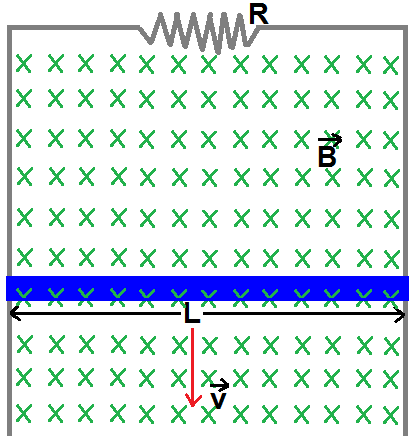# A sliding conductorA horizontal conducting rod of mass $m$ and length $L$ is free to slide on two vertical conducting rails as shown, where there is a magnetised​ field of intensity $B$ directed inside the screen and the resistance of the resistor is $R$. Find the distance travelled by the rod after $t$ seconds.

×